Two-Pole Next  |  Prev  |  Up  |  Top  |  Index  |  JOS Index  |  JOS Pubs  |  JOS Home  |  Search

### Two-Pole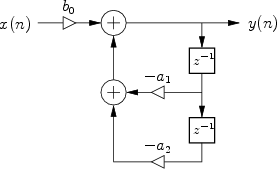The signal flow graph for the general two-pole filter is given in Fig.10.6. We proceed as usual with the general analysis steps to obtain the following: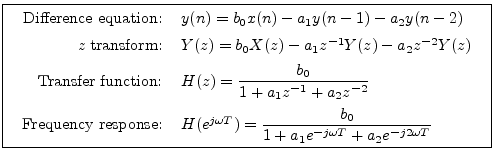The numerator of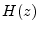is a constant, so there are no zeros other than two at the origin of the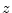plane.

The coefficients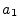and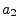are called the denominator coefficients, and they determine the two poles of. Using the quadratic formula, the poles are found to be located at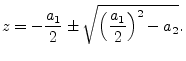When the coefficientsandare real (as we typically assume), the poles must be either real (when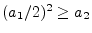) or form a complex conjugate pair (when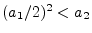).

When both poles are real, the two-pole can be analyzed simply as a cascade of two one-pole sections, as in the previous section. That is, one can multiply pointwise two magnitude plots such as Fig.10.5a, and add pointwise two phase plots such as Fig.10.5b.

When the poles are complex, they can be written as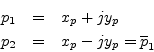since they must form a complex-conjugate pair whenandare real. we may express them in polar form as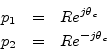where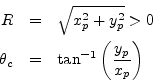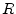is the pole radius, or distance from the origin in the-plane. As discussed in Chapter 8, we must have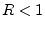for stability of the two-pole filter. The angles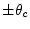are the pole's respective angles in theplane. The pole angle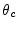corresponds to the pole frequency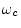via the relation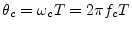where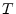denotes the sampling interval. See Chapter 8 for a discussion and examples of pole-zero plots in the complex-plane.

Ifis sufficiently large (but less than 1 for stability), the filter exhibits a resonance11.2 at radian frequency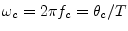. We may callor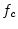the center frequency of the resonator. Note, however, that the resonance frequency is not usually the precise frequency of peak-gain in a two-pole resonator (see Fig.10.10 on page). The peak of the amplitude responses is usually a little different because each pole sits on the other's skirt,'' which is slanted. (See §10.1.5 and §10.6 for an elaboration of this point.)

Using polar form for the (complex) poles, the two-pole transfer function can be expressed as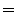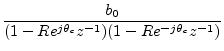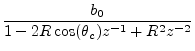(11.1)

Comparing this to the transfer function derived from the difference equation, we may identify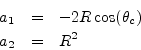The difference equation can be rewritten as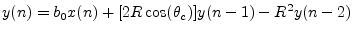(11.2)

Note that coefficientdepends only on the pole radius R (which determines damping) and is independent of the resonance frequency, whileis a function of both. As a result, we may retune the resonance frequency of the two-pole filter section by modifyingonly.

The gain at the resonant frequency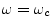, is found by substituting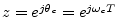into Eq. (10.1) to get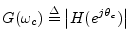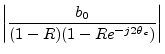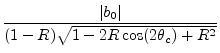(11.3)

See §10.6 for details on how the resonance gain (and peak gain) can be normalized as the tuning ofis varied in real time.

Since the radius of both poles is, we must havefor filter stability (see Chapter 8, §8.3 for the theory). The closeris to 1, the higher the gain at the resonant frequency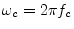. If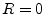, the filter degenerates to the form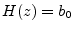, which is a nothing but a scale factor. We can say that when the two poles move to the origin of theplane, they are canceled by the two zeros there.

Subsections
Next  |  Prev  |  Up  |  Top  |  Index  |  JOS Index  |  JOS Pubs  |  JOS Home  |  Search

[How to cite this work] [Order a printed hardcopy]